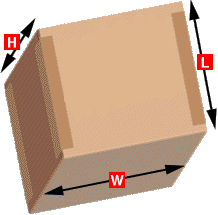Sample Dimensional Weight             Calculation W= 10"     H=12"    L=20" W x H x L  = 17.26 Lbs       139 Dimensional Weight is a standard formula used in the air-freight industry and considers package density when calculating shipping charges The calculation for dimensional weight considers the amount of space a package will take up on an aircraft in relation to the actual weight of the package. When calculating the airfreight costs, if the dimensional weight is more than the actual weight, the cost is based on the dimensional weight. Here's how to calculate dimensional weight of a package which is 10" Wide, 12" High and 20" Long. 1. Multiply the Length x Width x Height            10 x 12 x 20 = 2400 2. Divide this result (the "cubic size") by 139    2400/139 = 17.26 3. The result is the dimensional weight (in pounds)